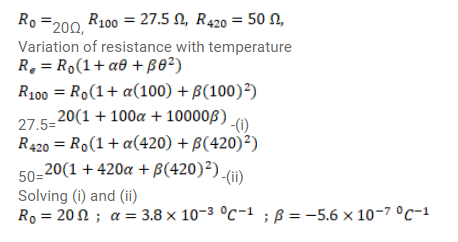A resistance thermometer reads $R=20.0 \Omega, 27.5 \Omega$ and $50.0 \Omega$ at the ice point $\left(0^{\circ} \mathrm{C}\right)$, the steam point ( $100^{\circ} \mathrm{C}$ ) and the zinc point $\left(420^{\circ} \mathrm{C}\right)$ respectively. Assuming that the resistance varies with the temperature as $R_{e}=R_{0}\left(1+\alpha \theta+\beta \theta^{2}\right)$, find the values of $R_{0}, \alpha$ and $\beta$. Here $\theta$ represents the temperature on Celsius scale.